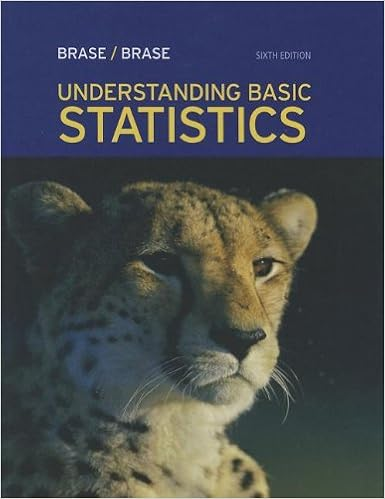# Students who visit the bookstore are given flyers

• Notes
• 3
• 100% (3) 3 out of 3 people found this document helpful

This preview shows page 1 - 3 out of 3 pages.

##### We have textbook solutions for you!
The document you are viewing contains questions related to this textbook.The document you are viewing contains questions related to this textbook.
Chapter 2 / Exercise 2
Understanding Basic Statistics
Brase/BraseExpert Verified
with the bookstore service and merchandise. Students who visit the bookstore are given flyers requesting that they visit a special website to answer questions for the survey.
##### We have textbook solutions for you!
The document you are viewing contains questions related to this textbook.The document you are viewing contains questions related to this textbook.
Chapter 2 / Exercise 2
Understanding Basic Statistics
Brase/BraseExpert Verified
d.A college cafeteria manager conducts a survey of 150 students to find out howsatisfied students are with the service at the newly renovated cafeteria. In order to get students using the cafeteria throughout the day, he randomly selects 50 students in the morning, 50 in the afternoon, and 50 in the evening. 5. Using the data in problem number 3, calculate/determine the following.a.average = _________b.standard deviation = _______c.Q1 = ______d.Median = _______e.Mode = _______f.Q3 = ______g.IQR = ______h.The data value that is 2.5 standard deviations below the mean _______i.10thpercentile = _______j.90thpercentile = _______6. Using the data in problem number 3, construct a histogram and boxplot. Label and scale both axes.7. The following table identifies the number of hours that a certain group of De Anza students work per weekNumber of hours student works per week:Major is in the Division:0-910-1415-19At least 20TOTALPSME201285Language Arts711108Business181722TOTALa.What is the probability that a randomly selected student of the group has a major in Business?b.What is the probability that a randomly selected student of the group either works from 0 to 9 hours a week or has a major in Language Arts?c.
•••